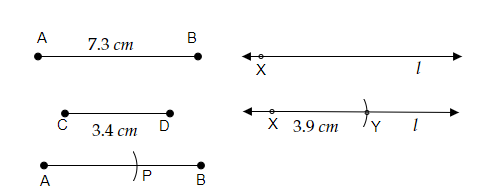# Given $\overline{\mathrm{AB}}$ of length $7.3 \mathrm{~cm}$ and $\overline{\mathrm{CD}}$ of length $3.4 \mathrm{~cm}$, construct a line segment $\overline{X Y}$ such that the length of $\overline{X Y}$ is equal to the difference between the lengths of $\overline{\mathrm{AB}}$ and $\overline{\mathrm{CD}}$. Verify by measurement.

Given:

Given $\overline{AB}$ of length $7.3\ cm$ and $\overline{CD}$ of length $3.4\ cm$.

To do:

We have to construct a line segment $\overline{XY}$ whose length is equal to the difference between the lengths of $\overline{AB}$ and $\overline{CD}$ and verify it by measurement.

Solution:Steps of construction:

(i) First let us take compasses and by pointing the pointer of the compasses at point C of the line segment CD let us take the length of the line segment CD with compasses.

(ii) Now, by placing the pointer of the compasses on point A of the line segment AB let us draw an arc and point it as P.

(iii) let us draw the line 'l' and mark a point X on it.

(iv) let us adjust the compasses up to the length of PB, by pointing the pointer of compasses on point X let us draw an arc on the line 'l' and point it as point Y.

(v) Therefore, The line segment $\overline{XY}$ is formed.

(vi) The difference between the lengths of $\overline{AB}$ and $\overline{CD}$ is $7.3\ cm - 3.4\ cm = 3.9\ cm$.

(vii) We can verify the measurement by placing the ruler's starting point on point X to point Y measures $3.9\ cm$.

Updated on: 10-Oct-2022

20 Views